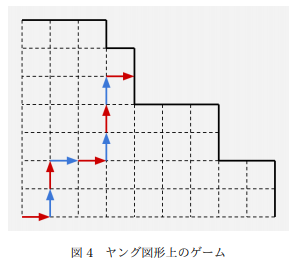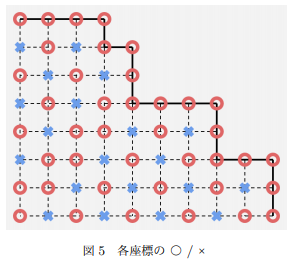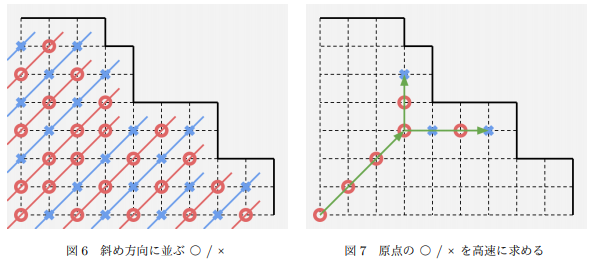5G时代下一个聚合的编程学习网

# AtCoder AGC002 简要题解

### E

• 假设操作一选择了 (x) 个元素，那么这 (x) 个元素不会因为操作而改变，一定会是最大的前 (x) 个元素（有相同的随便取）。### F

• 将每种颜色第一次出现的位置依次写下记为 (A)，第二次出现的位置依次写下记为 (B)，那么这样的原始序列合法当且仅当 (A, B) 离散化之后的序列完全相同！

[f_{i, j, k} = (n – i + 1) imes sumlimits_{x < j} sumlimits_{y < k – 1} f_{x, y} imes frac{((i – 1) imes n + 2 – k + 1) ^ {overline{n – 2}}}{(n – 2)!}
]

[f_{i, j} = f_{i – 1, j} + (n – j + 1) imes f_{i, j – 1} imes dbinom{n imes m – (j – 1) imes (n – 1) – i – 1}{n – 2}
]

GO!